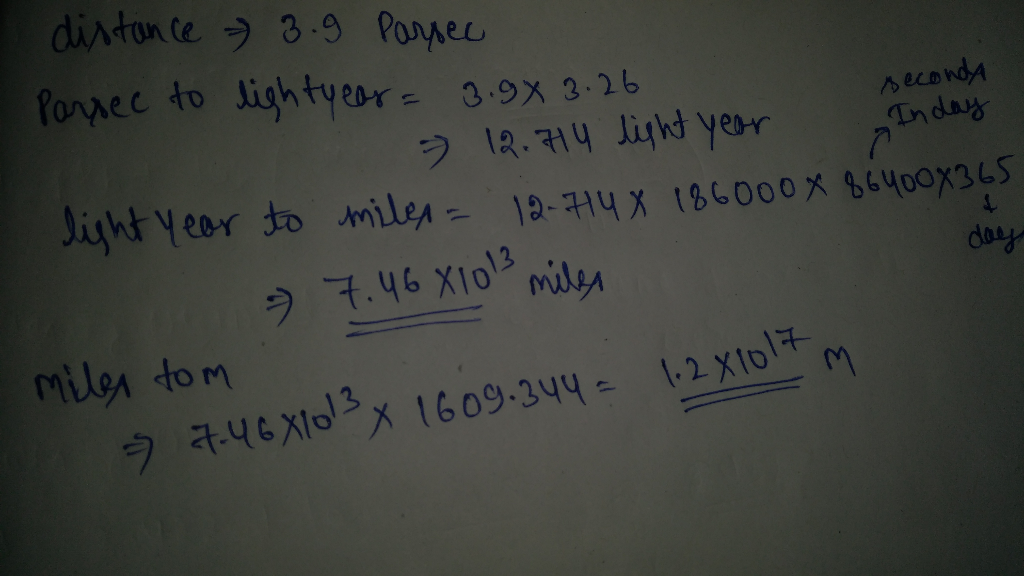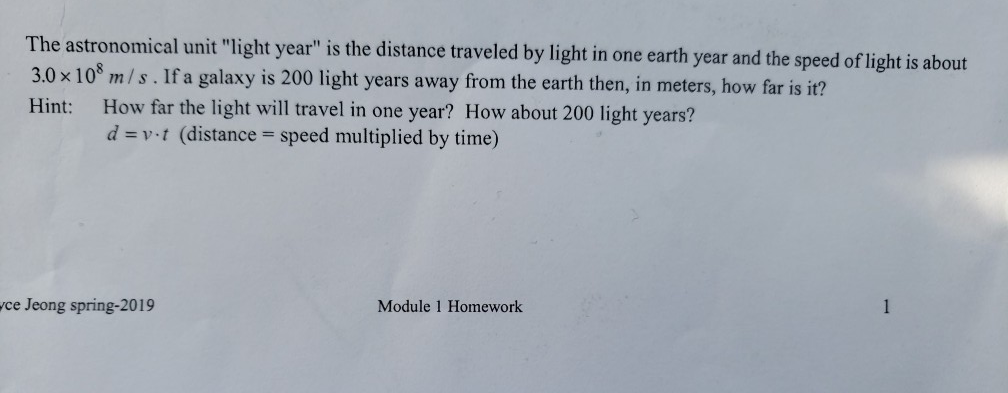Question

# A parsec is an astronomical unit of distance where 1 parsec = 3.26 light years (1...

A parsec is an astronomical unit of distance where 1 parsec = 3.26 light years (1 light year equals the distance traveled by light in one year). If the speed of light is 186,000 mi/s, calculate the distance in meters of an object that travels 3.9 parsecs.If you have any query please comment

If you satisfied with the solution please like it thankxx

#### Earn Coins

Coins can be redeemed for fabulous gifts.

Similar Homework Help Questions
• ### In solar Astronomy the standard unit of measurement for distance is the Astronomical Unit which is...

In solar Astronomy the standard unit of measurement for distance is the Astronomical Unit which is the average distance from the Earth to the Sun ( 1 1.5 10 ) 8 AU =  km . In stellar Astronomy the distances are so large, that the standard units of length are the Light Year (LY) or the Parsec. A light year is the distance traveled by light in one year, know that light travels 300000 km per second in empty...

• ### please and thank you The astronomical unit "light year" is the di 3.0x10* m/s. If a...please and thank you The astronomical unit "light year" is the di 3.0x10* m/s. If a galaxy is 200 light years away from the earth then, in meters, how far is it? Hint: How far the light will travel in one year? How about 200 light years? stance traveled by light in one earth year and the speed of light is about d- (distance speed multiplied by time) yce Jeong spring-2019 Module 1 Homework

• ### Easy physics

An astronomical unit (AU) is equal to the average distance from Earth to the Sun, about 92.9 × 106 mi. A parsec (pc) is the distanceat which a length of 1 AU would subtend an angle of exactly 1 second of arc (Figure 1-8). A light-year (ly) is the distance that light, traveling througha vacuum with a speed of 186000 mi/s, would cover in 1.0 year. Express the Earth–Sun distance in (a) parsecs and (b) light-years.

• ### Light Years

A "light-year" is the distance light (speed = 2.998 10^8 m/s) travels in one year.(a) How many meters are there in 7.00 light-years?1(b) An astronomical unit (AU) is the average distance from the Sun to Earth, 1.50 10^8 km. How many AU are there in 7.00 light-year?2(c) What is the speed of light in AU/h?3

• ### A certain spaceship has a speed of 13600 mi/hr. What is its speed in light-years/century? A...

A certain spaceship has a speed of 13600 mi/hr. What is its speed in light-years/century? A light-year is the distance that light travels in one year with a speed of 186,000 mi/s.

• ### light-year

a light-year is the distance light travels in one year (atspeed=2.998x108m/s).1) how many meters are there in 1.00 light-year?2) an astronomical unit (AU) is the average distance from thesun to earth, 1.50x 108 km. how manyAU are there in 1.00light-year?3) what is the speed of light in AU/h?

• ### A light-year is defined as the distance traveled by light in a vacuum during one tropical...

A light-year is defined as the distance traveled by light in a vacuum during one tropical year. How many light-years are in a parsec?

• ### An astronomical unit (AU) is the average distance of the Earth from the Sun, approximately 1.50 108 km

An astronomical unit (AU) is the average distance of the Earth from the Sun, approximately 1.50 108 km. The speed of light is about 3.0 108 m/s. Express the speed of light in terms of astronomical units per minute.

A "light-year" is the distance light (speed = 2.998 x 10^8 m/s) travels in one year.(a) How many meters are there in 10.0 light-years?(b) An astronomical unit (AU) is the average distance from the Sun to Earth, 1.50 x 10^8 km. How many AU are there in 10.0 light-year?(c) What is the speed of light in AU/h?

• ### a light year is the distance that light can travel in one year's time. If the...

a light year is the distance that light can travel in one year's time. If the speed of light is 3.0 x 10^8 m/s, how many meters are in a light day, i.e., the distance that light travels in one day?